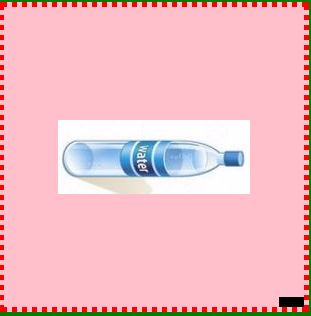# Canvas Images in HTML5

### Canvas images

There are three steps to draw the canvas images

They are as follow,

`context.drawImage(image, dx, dy);`
`context.drawImage(image, dx, dy, dw, dh);`
`context.drawimage(image, sx, sy, sw, sh, dx, dy, dw, dh);`

• dx- It represents value of  the x
• dy- Itrepresents value of  the y
• dw- It represnts value of the width
• dh- It reprents the value of the height
• sx- It represents the value of the source x
• sy- It represents the value of the source y
• sw- It represents the value of the source width
• sh- It represents the value of the source height

### Draw image

Canvas images uses the syntax as `drawimage()` method to draw the image or to increase the size of the image

``` <canvas id="myCanvas" width="500" height="200"></canvas>
<script>
var canvas = document.getElementById('myCanvas');
var context = canvas.getContext('2d');
var imageObj = new Image();

context.drawImage(imageObj, 20, 40);
};
imageObj.src = "http://techfunda.com/HTPictures/Generics/1-635716259621984414.jpg";
</script>
```

In the above code snippet we have defined the images in canvas, We have canvas id as `"mycanvas"` and width as `"500"` and height as` "200`

• var canvas = document.getElementById('myCanvas'); - It defines the var canvas as document.getElementById as"myCanvas"
• var context as canvas .getcontext("2d"); - It defines the context value to canvas
• var imageObj = new Image(); - It defines the var imageobj to new image()
• imageObj.onload = function () - It defines the function of the imageobj as onload
• `context.drawImage(imageObj, 20, 40);` - It defines the image with x, y positions

In the last step we have defined the url of the image

outputImage size

It defines the size of the image with the width and height

``` <canvas id="myCanvas" width="278" height="400"></canvas>
<script>
var canvas = document.getElementById('myCanvas');
var context = canvas.getContext('2d');

var x = 20;
var y = 15;
var width = "200";
var height = "350";
var imageObj = new Image();
context.drawImage(imageObj, x, y, width, height);
};
imageObj.src = "http://techfunda.com/HTPictures/Generics/1-635716259621984414.jpg";
</script>
```

Image-size

In the above code snippet we have defined the images in canvas, We have canvas id as `"mycanvas"` and width as` "278`" and height as `"400"`

• var canvas = document.getElementById('myCanvas'); - It defines the var canvas as document.getElementById as`"myCanvas"`
• var context as canvas .getcontext("2d"); - It defines the context value to canvas
• var(x,y, width, height) - (20,15, 200, 350)
• var imageObj = new Image(); - It defines the var imageobj to new image()
• imageObj.onload = function () - It defines the function of the imageobj as onload
• `context.drawImage(imageObj, x, y, width, height); `- It defines the image with x, y positions

In the last step we have defined the with the url of the image

output### Rotating image by 90 degrees

It is used to rotate the image

``` <canvas id="canvas" width=300 height=300></canvas>
<script>
var canvas = document.getElementById("canvas");
var ctx = canvas.getContext("2d");

var img = new Image();
img.src = "http://techfunda.com/HTPictures/Generics/5-635898849972807270.JPG";

function start() {
ctx.translate(150, 150);
ctx.rotate(Math.PI / 2);
ctx.drawImage(img, -img.width / 2, -img.height / 2);
ctx.rotate(-Math.PI / 2);
ctx.translate(-canvas.width / 2, -canvas.height / 2);
ctx.fillRect(0, 0, 25, 10);
}
</script>
```

Rotating image

• In the above code snippet we have defined to rotate the image towards 90 degrees by using canvas, we are having canvas as width and height with 300
• var canvas = document.getElementById("canvas"); - we are declaring canvas to the id
• var ctx = canvas.getContext("2d"); - we are declaring the context to the canvas
• In the nextline we are declaring image to the new image with the given image source
• ctx.translate(150, 150); - It is used to set the image to the center of the canvas
• ctx.rotate(Math.PI / 2); - It is used to rotate the image in the canvas
• ctx.drawImage(img, -img.width / 2, -img.height / 2); - It defines the values of the image with(x, y) values
• ctx.rotate(-Math.PI / 2); - It rotates the image in the canvas
• ctx.translate(-canvas.width / 2, -canvas.height / 2); - It sets the image center in the canvas
• ctx.fillRect(0, 0, 25, 10); - It defines the values of the (x, y, width, height);

outputViews: 4313 | Post Order: 38